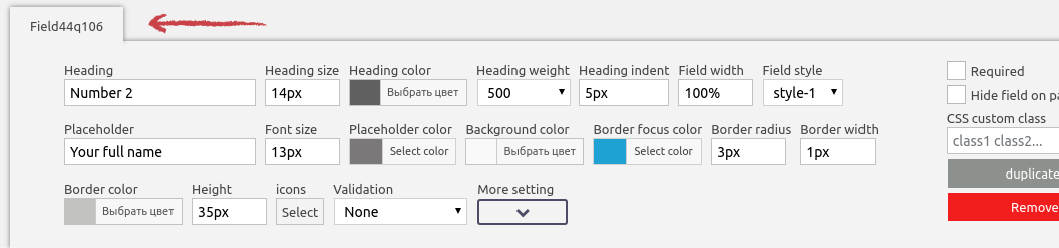## How to use math formulas

In order to create a calculator you need to add the ‘total’ field:Simple example

In the “total” field editor we see two text areas, the first contains a formula in which you specify field identifiers and mathematical operators. Basic operators are allowed in math formulas: + – / * ( )

The screenshot below shows an example of summing the value of two fields in your form.Id of any field you can see in the editor:In addition to id fields in the formula, you can specify any numbers, fractional numbers are indicated by a dot, for example 10.5

## Field values ​​for calculator

If you need to create a calculator, then the form fields must be assigned a value for calculation

Fields such as ‘input’ or ‘textarea’ do not need to be assigned values, since their value is their content

You need to assign values ​​to the ‘checkboxes’ and ‘dropdowns’. For both, this value is configured equally.

In the text field, before the option or flag is named, write number==. An example you can see in the screenshot:In this example, we have two checkboxes ‘item-1’ and ‘item-2’, which have values ​​for the calculator ‘100’ and ‘300’.

### Note

If you need real examples of calculators, you can see them in the demo. You can also transfer the calculator from the demo to your site through the import / export of forms.